# Making maps using geofi-package

#### 2022-10-23

Installation

`geofi` can be installed from CRAN using

``````# install from CRAN
install.packages("geofi")

# Install development version from GitHub
remotes::install_github("ropengov/geofi")``````
``````# Let's first create a function that checks if the suggested
# packages are available
check_namespaces <- function(pkgs){
return(all(unlist(sapply(pkgs, requireNamespace,quietly = TRUE))))
}``````

This vignettes gives an overview of different options for creating maps in R using the data from `geofi`-package. Vignette is divided in three sections: R-packages for static maps, static maps using ggplot2 and interactive maps. But we begin with the datasets we want to plot.

If you want more detailed explanation of how to plot `sf`-objects take a look at vignette 5. Plotting Simple Features.

## Datasets

Lets start with latest municipality division from `get_municipalities()` with is a POLYGON data and with POINT data of `municipality_central_localities` that is shipped with the package.

``````library(geofi)
polygon <- get_municipalities(year = 2021, scale = 4500)
point <- geofi::municipality_central_localities
# municipality code into integer
point\$municipality_code <- as.integer(point\$kuntatunnus)
library(sf) # for spatial data operations later``````

They both come in same CRS EPSG:3067 and can be plotted together without any further manipulation.

## R-packages for static maps

There are two main technologies for creating static graphics in R: base and ggplot2. Both can be used to plot spatial data ie. to create maps. In addition, tmap : thematic maps in R is a great tool if you want to dig deeper into cartography in R.

base

``````# dev.off()
plot(st_geometry(polygon["municipality_code"]))
plot(st_geometry(point["municipality_code"]), add = TRUE, color = "black")``````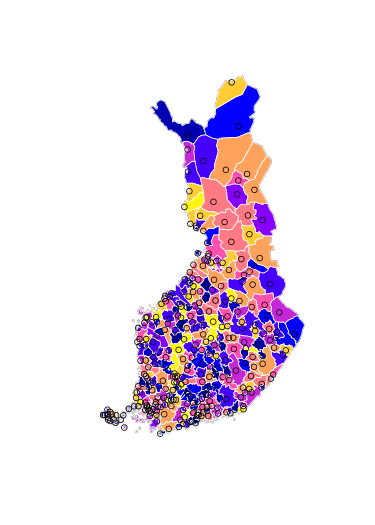ggplot2

``````libs <- c("ggplot2")
if (check_namespaces(pkgs = libs)) {
library(ggplot2)
ggplot() +
geom_sf(data = polygon, aes(fill = municipality_code)) +
geom_sf(data = point)
} else {
message("One or more of the following packages is not available: ",
paste(libs, collapse = ", "))
}``````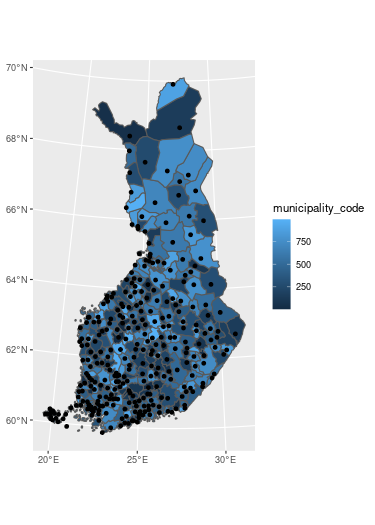tmap

`tmap` is a versatile library for creating static thematic maps in R. It supports `sf`-class objects and is fully compatible with geospatial data available through `geofi`.

As I am only fluent in using `ggplot2` the the more complex examples are using `ggplot2`-package.

## Static maps using ggplot2

`ggplot2`-packages has three `sf`-class spesific functions: `geom_sf` plotting for points, lines and polygons, and `geom_sf_text` and `geom_sf_label` for labeling the maps. In the following examples we are using the Uusimaa region in Southern Finland.

``````libs <- c("ggplot2")
if (check_namespaces(pkgs = libs)) {
library(dplyr)
polygon_uusimaa <- polygon %>% filter(maakunta_name_fi %in% "Uusimaa")
point_uusimaa <- point %>% filter(municipality_code %in% polygon_uusimaa\$municipality_code)
ggplot() +
theme_light() +
geom_sf(data = polygon_uusimaa, alpha = .3) +
geom_sf(data = point_uusimaa) +
geom_sf_text(data = point_uusimaa, aes(label = teksti))
} else {
message("One or more of the following packages is not available: ",
paste(libs, collapse = ", "))
}``````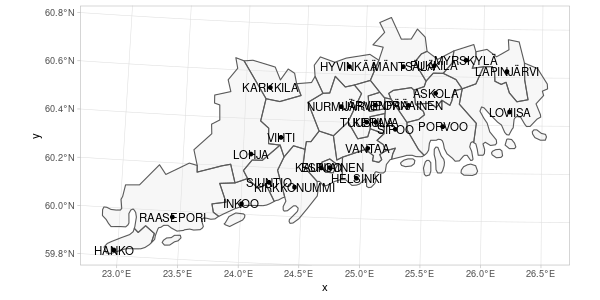### Label overlapping

`geom_sf_label` or `geom_sf_text` cannot control the overlapping of labels which is a common issue when mapping objects of various shapes and sizes. With `ggrepel` you can solve the problem though it requires a bit of spatial data processing with `sf`-package.

``````libs <- c("ggplot2")
if (check_namespaces(pkgs = libs)) {
ggplot() +
theme_light() +
geom_sf(data = polygon_uusimaa, alpha = .3) +
geom_sf(data = point_uusimaa) +
ggrepel::geom_text_repel(data = point_uusimaa %>%
sf::st_set_geometry(NULL) %>%
bind_cols(point_uusimaa %>%
sf::st_centroid() %>%
sf::st_coordinates() %>% as_tibble()),
aes(label = teksti, x = X, y = Y))
} else {
message("One or more of the following packages is not available: ",
paste(libs, collapse = ", "))
}``````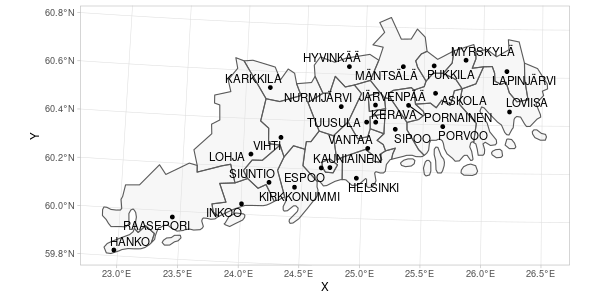### Faceting

If want to present multiple variables of same regions you can use facets.

### Facetting and combining maps

Facetting is a useful way to present data on multiple variables covering the same region. This is useful approach if you have, lets say, data on same indicator from two different time points and you want to have separate maps for separate times points, but have a shared scale. Below I create a random data for two year titled `population` and plot the data using `facet_wrap()`-function.

``````pop_data <- bind_rows(
tibble(
municipality_code = polygon\$municipality_code
) %>%
mutate(population = rnorm(n = nrow(.), mean = 2000, sd = 250),
time = 2020),
tibble(
municipality_code = polygon\$municipality_code
) %>%
mutate(population = rnorm(n = nrow(.), mean = 2000, sd = 250),
time = 2021)
)
pop_data
#> # A tibble: 618 × 3
#>    municipality_code population  time
#>                <int>      <dbl> <dbl>
#>  1                 5      1854.  2020
#>  2                 9      2199.  2020
#>  3                10      1984.  2020
#>  4                16      2116.  2020
#>  5                18      2144.  2020
#>  6                19      1784.  2020
#>  7                20      1857.  2020
#>  8                35      1691.  2020
#>  9                43      2458.  2020
#> 10                46      2148.  2020
#> # … with 608 more rows``````
``````pop_map <- right_join(polygon, pop_data)

libs <- c("ggplot2")
if (check_namespaces(pkgs = libs)) {
ggplot(pop_map,
aes(fill = population)) +
geom_sf() +
facet_grid(~time)
} else {
message("One or more of the following packages is not available: ",
paste(libs, collapse = ", "))
}``````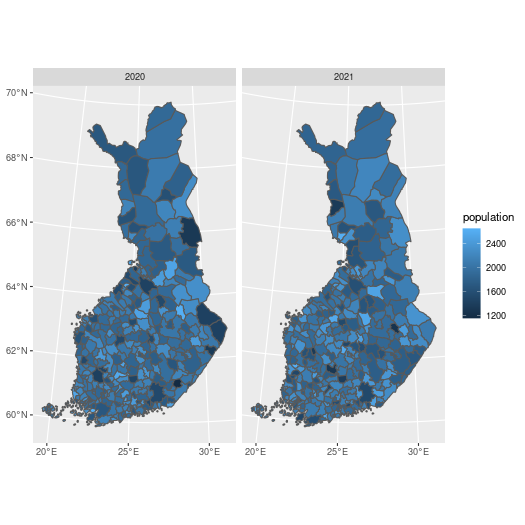However, often the indicators you want to compare either have different values (shared scale not ideal), are aggregated differently or cover non-overlapping geographic region. The you may find patchwork useful as in the example below.

``````libs <- c("ggplot2","patchwork")
if (check_namespaces(pkgs = libs)) {
library(patchwork)
p_municipalities <- ggplot(polygon, aes(fill = municipality_code)) +
geom_sf() +
theme(legend.position = "top")
p_regions <- ggplot(polygon %>% count(maakunta_code), aes(fill = maakunta_code)) +
geom_sf() +
theme(legend.position = "top")
p_uusimaa <- ggplot(polygon_uusimaa, aes(fill = municipality_code)) +
geom_sf() +
theme(legend.position = "top")

(p_municipalities | p_regions) /
p_uusimaa + plot_layout(nrow = 2, heights = c(1,0.6)) +
plot_annotation(title = "Combining multiple maps into a single (gg)plot")
} else {
message("One or more of the following packages is not available: ",
paste(libs, collapse = ", "))
}``````